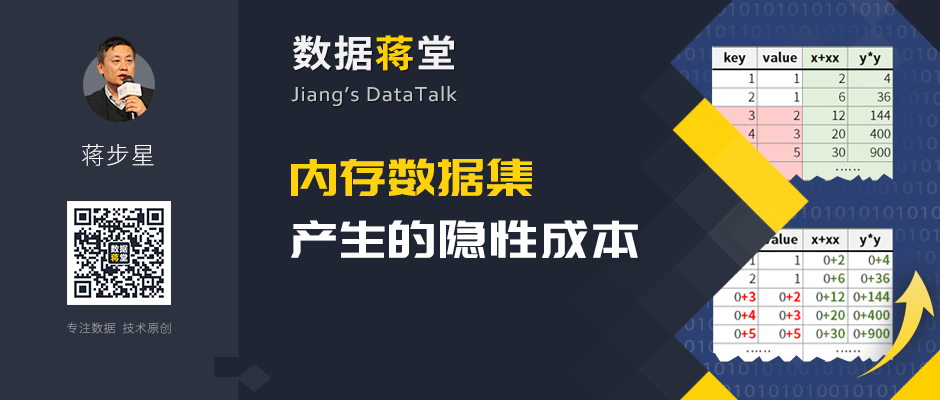# 内存数据集产生的隐性成本A B 1 =create(x,xx) 2 for 100 >A1.insert(0,A2,A2*A2)

 A B 1 =100.new(~:x,~*~:xx)

 A B 1 =create(key,value) 2 >a=0 >b=1 3 for 20 >A1.insert(0,A3,b) 4 >b=a+b,a=b-a

 A B 1 =20.new(0:key,0:value) 2 >a=0 >b=1 3 for A1 >A3.key=#A3,A3.value=b 4 >b=a+b,a=b-a

 A B 3 =A1.derive(x+xx:y) =A3.derive(y*y:yy)

 A B 3 =A1.derive(x+xx:y,0:yy) >A3.run(yy=y*y)

 A B 1 =db.query("select month,amount from T order by month) 2 =A1.derive(0:acc) >a=0 3 for A1 >A3.acc=(a=a+A3.amount)

 A B 1 =db.query("select month,amount,0 as acc from T order by month) >a=0 2 for A1 >A2.acc=(a=a+A2.amount)